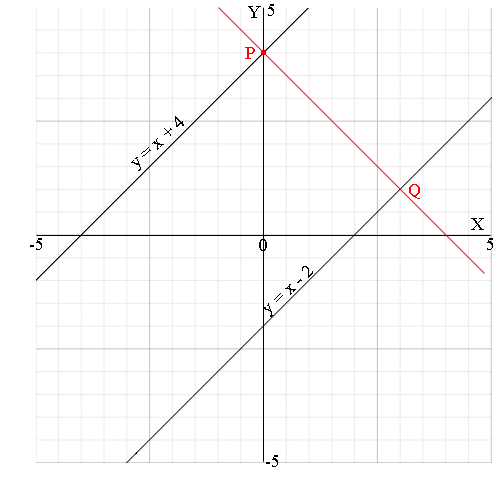SEARCH HOMEMath Central Quandaries & QueriesQuestion from christianah, a student: Two parallel lines have the equations y=x+4 and y=x-2.Two additional lines are drawn to form a square.What is the side length of the square?Write possible equations for the additional lines. Explain and justify your reasoning.Christianah,

I can help get you started. First I sketched a graph of the two given lines.Let P be the point where the line y = x + 4 intersects the Y-axis.

What are the coordinates of P?

Draw the line through P that is perpendicular to y = x + 4. This is going to form the third side of the square. It intersects y = x - 2 at Q. The line y = x + 4 has slope 1.

What is the slope of the line through P and Q?
What is the equation of this line?
What are the coordinates of Q?

The side length of the square is the distance from P to Q. You can find this using the distance formula or by using Pythagoras theorem directly.

I hope this helps. Write back if you need more assistance.

PennyMath Central is supported by the University of Regina and The Pacific Institute for the Mathematical Sciences.